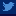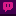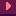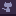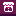Back Bresenham algorithm

Bresenham is a quite common algorithm I actually discovered only a few months ago. And it proved to be incredibly useful for many purposes.

This algorithm is basically used to draw a line between 2 points in a grid based space (ie. pixels). The result is a pixel perfect line, which is cool.

But this algorithm has also many other interesting usages:

• line of sight
• pathfinding optimization
• pathfinding smoothing
• field of vision (cone)
• lighting

## Implementation

You can check my Haxe implementation from my GitHub libs:

Here is the implementation I use in Haxe:

``````function getLine(x0:Int, y0:Int, x1:Int, y1:Int) : Array<{x:Int, y:Int}> {
var pts = [];
var swapXY = fastAbs( y1 - y0 ) > fastAbs( x1 - x0 );
var tmp : Int;
if ( swapXY ) {
// swap x and y
tmp = x0; x0 = y0; y0 = tmp; // swap x0 and y0
tmp = x1; x1 = y1; y1 = tmp; // swap x1 and y1
}

if ( x0 > x1 ) {
// make sure x0 < x1
tmp = x0; x0 = x1; x1 = tmp; // swap x0 and x1
tmp = y0; y0 = y1; y1 = tmp; // swap y0 and y1
}

var deltax = x1 - x0;
var deltay = fastFloor( fastAbs( y1 - y0 ) );
var error = fastFloor( deltax / 2 );
var y = y0;
var ystep = if ( y0 < y1 ) 1 else -1;
if( swapXY )
// Y / X
for ( x in x0 ... x1+1 ) {
pts.push({x:y, y:x});
error -= deltay;
if ( error < 0 ) {
y = y + ystep;
error = error + deltax;
}
}
else
// X / Y
for ( x in x0 ... x1+1 ) {
pts.push({x:x, y:y});
error -= deltay;
if ( error < 0 ) {
y = y + ystep;
error = error + deltax;
}
}
return pts;
}``````

Note that `fastAbs` and `fastFloor` are fast implementations of absolute and floor methods:

``````static inline function fastAbs(v:Int) : Int {
return (v ^ (v >> 31)) - (v >> 31);
}

static inline function fastFloor(v:Float) : Int {
return Std.int(v); // actually it's more "truncate" than "round to 0"
}``````

A few things to know about Bresenham algorithm:

• it’s simple to implement, quite fast and efficient,
• I’ve moved the `if( swapXY )` outside of the loop for slightly faster results (only on very large number of calls),
• the Array memory allocation (ie. `var pts = []`) is not free. You may want to specialize this function to your needs instead of pushing points into an Array.
• the array order can vary. This is really important! It means that the array returned can be either from x0,y0 to x1,y1, or the contrary ; it depends on the angle.

I encourage you to read the normal implementation of Bresenham on Wikipedia, especially if you plan to specialize its implementation to your needs. You will also find other interesting optimizations there.

So. What can we do with this algorithm?

## Use cases

### Artificial intelligence

When you write an AI for your monsters, you will often want to answer 2 basic questions:

• is the mob close to the player (basically, checking distances)
• does he see the player?

The second question is easily answered using a Bresenham algorithm to check if there is anything blocking sight between the monster and the player.

``````function checkLine(x0:Int, y0:Int, x1:Int, y1:Int, rayCanPass:Int->Int->Bool) {
var swapXY = fastAbs( y1 - y0 ) > fastAbs( x1 - x0 );
var tmp : Int;
if ( swapXY ) {
// swap x and y
tmp = x0; x0 = y0; y0 = tmp; // swap x0 and y0
tmp = x1; x1 = y1; y1 = tmp; // swap x1 and y1
}

if ( x0 > x1 ) {
// make sure x0 < x1
tmp = x0; x0 = x1; x1 = tmp; // swap x0 and x1
tmp = y0; y0 = y1; y1 = tmp; // swap y0 and y1
}

var deltax = x1 - x0;
var deltay = fastFloor( fastAbs( y1 - y0 ) );
var error = fastFloor( deltax / 2 );
var y = y0;
var ystep = if ( y0 < y1 ) 1 else -1;

if( swapXY )
// Y / X
for ( x in x0 ... x1+1 ) {
if( !rayCanPass(y,x) )
return false;

error -= deltay;
if ( error < 0 ) {
y = y + ystep;
error = error + deltax;
}
}
else
// X / Y
for ( x in x0 ... x1+1 ) {
if( !rayCanPass(x,y) )
return false;

error -= deltay;
if ( error < 0 ) {
y = y + ystep;
error = error + deltax;
}
}
return true;
}``````

This version doesn’t returns an array of points, it just run a given function (rayCanPass) on every points in the line and if this given function returns false, then the whole checkLine returns false and stops.

Example:

``````checkLine(
mob.x, mob.y,
player.x, player.y,
function(x,y) return collisionMap[x][y] == false
);``````

Neat and quite fast, especially because the loop stops if there is an obstacle. Note that function calls in Flash are expensive if you need to loop many times on checkLine, for example.

### Pathfinding optimization

When you write pathfinding algorithms (like A-star), you know by nature that calling them will be expensive in real-time games. So every time you can avoid a call to your pathfinder, it’s better to do so.

Using the previous example, if you can answer the question “does this mob see the player”, you can often skip useless pathfinding calls.

### Path smoothing

Many pathfinding algorithms return the complete list of points between START and END points, but also stick to the grid.

Bresenham can be used to very easily “smooth” this result. What you have to do is:

• set a point called REF which is equal to START to begin with,
• check if the REF points “sees” the 3rd point of the path. If so, remove 2nd point because it is basically useless.
• repeat by checking if REF points sees 4th point, etc.
• If the REF point cannot see a given point, then the previous point is useful and should be kept. In such a case, the REF is now that previous point and you can repeat the algorithm with the next points.

This way, you clean the path, only keeping successive points that see each other, and removing useless intermediaries.

### Field of vision (cone)

It’s easy to implement a cone of vision like in Metal Gear Solid or Commando.

To check if an enemy sees the player:

• check the distance,
• if in range, calculate the angle between the enemy and the player (Math.atan2)
• if the angular distance between this angle and the current direction of the enemy is less than the cone range / 2, run a Bresenham check
• if the player is not hidden by something… ALARM!

Note that if your game has diagonal walls, the basic bresenham algorithm will “see” through them.

### Lighting

If you iterate all points within range around, say, a Torch in rogue-like game, you can use Bresenham again to check if the Torch sees this particular point. If so, you can lighten up this cell, and the intensity of this lighting is one minus the distance to the torch divided by the max range.

``var intensity = 1 - dist / maxDist; // 0=no light, 1=full light``

It is incredibly expensive in realtime, because you have to iterate every single points around each torch to calculate your lightmap. But if you don’t need realtime, for example, if the torches don’t move dynamically, you can precalculate your lightings at the beginning of the game and the cost becomes negligible. And it’s really simple to implement.

``````for (dx in -radius...radius+1)
var x = source.x + dx;
var y = source.y + dy;

var d = distance(source.x, source.y, x, y);
if( d<=radius && checkLine(source.x,source.y, x,y, hasNoCollision) ){
var intensity = 1 - d/radius;
// update your lightmap, draw, ...
}
}``````

You can still have one dynamic lighting for your player, like in a roguelike, or only recalculate lighting when the player cell changes (ie. when he moves).

There is lots of room for optimizations here, but it all depends on your particular needs.

### Pixel perfect circles

Bresenham algorithm is used to draw lines, but it can also draw nice circles. Actually, the author is not Bresenham himself, but the approach is heavily inspired.

It’s not as useful as the line thing, but it can be useful and it’s easy to implement. Read more about it on Wikipedia.

You can fix this by adding a few extra points during a check: each time the line “breaks” (ie. error < 0), you can check additional points around this break. The result is thicker diagonals, without affecting horizontal and vertical lines.

If you have any question, please leave them in the comments :)

1.:

Ok, so i just posted a comment below, and I see no edit button nor reply, so im writing a new comment, apparently Bresenham's algorythm is kinda like 1000x more efficient than mine(i mean, seriously, i thought i had calculated time wrong) and thats without the fastAbs() and fastFloor() soo, ima update my algorythm, you can see it at https://github.com/LucasRo7/Base-Project(its under src/com/LS7/core/Renderer.java in the drawLine() method)

July 23, 2016 at 07:52
2.:

My line method is not as efficient as Bresenham's, it uses the direction, loops through the distance of the 2 points, get the sin and cosine, do some more math and then draws on the screen, I never really needed a better algorithm(since I use java and it is fast enough), but i'm gonna try to use Bresenham's algorithm and compare it to mine and probably even update my lighting engine,wich is already fast even with my algorithm '-'

July 23, 2016 at 07:25
3.:

Can I implement Breseham RayCast algorithm in every A* Pathfinding algorithm? I have to make RayCasting, but I realy don't know where to start.

June 12, 2016 at 16:42
4.:

hi Sébastien,

Could you please share the source code of lighting flash with me? I'm sure it's fairly simple but as I'm just learning Haxe and game development, it would be nice to see any/every code snippet.

Thank you,
Mark

August 26, 2014 at 17:19
5.:

I too ran into the problem with diagonals, and solved it by writing a routine that took 1 vertical or horizontal step at a time, but never a diagonal step. That way, it would hit one of the two walls and not be able to get through the diagonal “hole”. It's pretty short, at 18 lines; see draw_line in http://www.redblobgames.com/pathfinding/grids/diagrams.ts . I should probably clean this up and publish it somewhere.

May 10, 2014 at 04:07
6.:

Have you thought about using the pixel traversal algorithm from pages 2-3 in the following paper? http://www.cse.yorku.ca/~amana/research/grid.pdf

It's similar to Bresenham, but it steps over all the pixels the ray/line touches even by a little bit, which might be more suited to this use case than the more visually-oriented Bresenham. It also more than likely fixes your problem with diagonals, as it only steps in one dimension at a time. I haven't looked into your specific implementation, but from the first image it seems like that's not the case.

I'd be interested in hearing your thoughts on it, thanks!

May 8, 2014 at 20:00
7.:

Cool set of techniques.

BTW to speed up pathfinding even more on a grid, you can preprocess the line of sight calculations. First, observe that on a grid, all of the *useful* intermediaries in your path smoothing algorithm occur at exterior corners (interior corners don't matter). By preprocessing the grid, we can find all of these exterior corners, and then perform pathfinding only on those points. So pathfinding runs much faster *and* you get smoother paths. Yay!

I plan to write this up in more detail but until then I have this interactive demo: http://www.redblobgames.com/pathfinding/grids/algorithms.html

April 29, 2014 at 17:46
•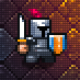:

This is an excellent idea =) I will definitely implement that on my AStar lib, thanks!
By the way, many of the articles you posted on your blog are references to me (especially the one on hexagons). Thanks for that too!

April 29, 2014 at 18:32
8.:

Wow, really nice article! The path smoothing is really clever… I tried to reproduce the lighting in c++, here is the result: http://www.youtube.com/watch?v=gL3tQRP1rEw&feature=youtu.be

Thanks for having shared this Bresenham magic! ^^

November 27, 2013 at 21:45
•:

Hi!
I'm glad you liked it that much. Well done for your implementation, it seems to work like a charm :)

November 27, 2013 at 22:47
9.:

Very nice article and extensive on Bresenham algorithm! A lot of useful tips, congratulations!

Can you make your lighting naive approach source code available? I would love to play with it!

Thank you, I despaired not to see you around. I'm happy you are back here :)

November 7, 2013 at 18:19
10.:

great article…. i'd never heard of this before, but it seems so useful… thanks…
and kudos on your games… they all look incredible and have a super art style as well!
i played green witch a bit, and liked the simple controls…. really works well.

November 4, 2013 at 18:54
•:

Thanks ^^

November 5, 2013 at 21:48
11.:

Nice article ! I discovered also Bresenham's algorithm not so long ago and used it to create a line in 3D space (yes, pixel perfect line in 3D) to arrange blocks automatically.
I'm not familiar enough with current version Flash, isn't there raycast ?

November 4, 2013 at 09:54
•:

Nope, you have to implement it by yourself.

November 4, 2013 at 12:14
12.:

Fantastic article. Would love to see some examples/techniques for "fat rays" (raycasting where rays have a specific thickness) and maybe an example of cone line of sight (angular distance can be tricky to get right sometimes).

November 3, 2013 at 12:52
13.:

Looks like a nice detailed article. I will someday, make sense of this! :P Right now, I'm just getting basic ideas of gaming algorithms, not how to use them.
Even though I have low info, you article explains it points pretty well!

November 3, 2013 at 12:16
•:

You only need to draw/cast as much of a line as you want.Let's say you're using ray casting to sialtmue a gun shot. The length(amount you draw) of the ray would be the max distance the bullet can travel.The best way to determine how much to draw is to use it, and play around with the distance until you find a good amount.Since it up to programmer to decide how much to draw/cast , how can it make sure 100% percent all objects will be checked?(The old method to traversal all objects in the stage can make sure every collided object be checked)

January 4, 2014 at 13:00
14.:

Nice article. A question, is Bresenham lineOfSight algorithm commutative ? Does checkLine(x0, y0, x1, y1, function()end) returns the same as checkLine(x1, y1, x0, y0, function()end) ?

Regards,
Roland.

November 3, 2013 at 08:49
•:

Hi,

No the base algorithm isn't commutative and will sometime return different results, depending on the order.

November 3, 2013 at 11:00
•:

Well, I was expecting that. It bugged me a lot when I was working on similar things lately: path smoothing using ThetA* algorithm (https://github.com/Yonaba/Jumper/blob/master/jumper/search/thetastar.lua#L18).

November 5, 2013 at 07:46
•:

Note: it's actually possible to make the algorithm commutative, by sorting the points given or adding extra points where the line "breaks" (ie. when error<0).

November 5, 2013 at 21:47
•:

Wow this looks awesome for colsliion detection that always is a pain! There is a plan to port these class in Haxe (nme) someday? that would be beautiful because we can use these colsliion method to makes game not only in flash but also mobile etc..nAnyway great job!

January 5, 2014 at 01:54